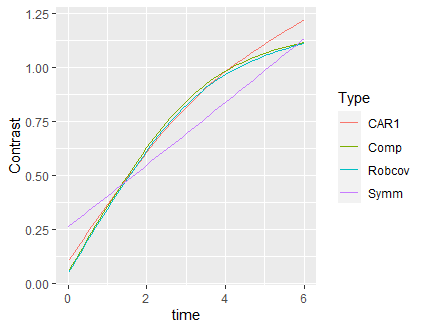# Robust covariance matrix estimation approach for serial data

Frank Harrell’s BBR lists five possible – valid and non-obsolete – approaches analyzing serial data (Chapter 15):

• generalized least squares
• mixed effects models
• Bayesian hierarchical models
• generalized estimating equations
• using appropriate summary measures

However, I can think of a further option: using the usual methods, but with cluster-robust covariance matrix, with clusters set as the observations from the same unit. The question is simply: is it a valid approach to analyze serial data?

It is very-very similar to GLS, with the exception that it relaxes one of the major limitations of GLS, that BBR also emphasizes, namely, that the response needs to be continuous and have a (conditional) normal distribution. With cluster-robust standard errors, any method can be used; `robcov` in `rms` also supports e.g. `lrm`, so we could immediately use this approach to model binary or ordinal outcome. And cluster-robust covariance matrix means – in my understanding – that practically any intra-individual correlation structure will be handled (especially if we have a large sample), so we don’t even have to “figure out” the appropriate correlation structure as with GLS.

Here is an illustration using the case study of BBR 15.3.1:

``````library(rms)
library(nlme)
library(data.table)
library(ggplot2)

d <- csv.get(paste('http://biostat.mc.vanderbilt.edu',
'dupontwd/wddtext/data/11.2.Long.Isoproterenol.csv',
sep='/'))
d <- upData(d, keep = c('id', 'dose', 'race', 'fbf'),
race = factor (race , 1:2 , c('white', 'black')),
labels = c(fbf='Forearm Blood Flow'),
units = c(fbf='ml/min/dl'))
d <-  data.table(d)
setkey(d, id, race)
d\$time <- match(d\$dose, c(0, 10, 20, 60, 150 , 300 , 400) ) - 1

fitCAR1 <- Gls(log(fbf) ~ rcs(time,3) * race + log(dose + 1), data = d,
correlation = corCAR1( form = ~ time | id))
fitCompSymm <- Gls(log(fbf) ~ rcs(time,3) * race + log(dose + 1), data = d,
correlation = corCompSymm( form = ~ time | id))
fitSymm <- Gls(log(fbf) ~ rcs(time,3) * race + log(dose + 1), data = d,
correlation = corSymm( form = ~ 1 | id))
fitRobcov <- robcov(ols(log(fbf) ~rcs(time,3) * race + log(dose + 1),
data = d, x = TRUE), d\$id)

res <- rbindlist(setNames(lapply(
list(fitCAR1, fitCompSymm, fitSymm, fitRobcov),
function(fit)
with(contrast(fit, list(time = seq(0, 6, by = 0.1), race = "white"),
list(time = seq(0, 6, by = 0.1), race = "black")),
data.table(time, Contrast, Lower, Upper))),
c("CAR1", "CompSymm", "Symm", "Robcov")),
idcol = "Type")

ggplot(res, aes(x = time, y = Contrast, color = Type)) + geom_line()
``````I slightly modified the example, as if my primary analysis question was the – possibly race-dependent – evolution of `fbf` over time. I hope it is correct in the above way.

(Again, the major point here is that if this robust covariance estimation approach is feasible, then it could be extended totally directly to non-normal responses, such as a binary outcome, which GLS can’t handle.)

2 Likes

This is exceptionally helpful Tamas. You are right the cluster sandwich covariance correction is widely applicable (in terms of types of models) and quick. I’ve done some limited situations with within-subject AR(1)-type correlation and the robust standard error estimate, while not perfect, is acceptable in accuracy. Here are some drawbacks:

• when using a working independence model as you are doing the cluster sizes are not allowed to vary very much across subjects
• it works best with a large number of small clusters (just as the cluster bootstrap does)
• it is very non-robust to dropouts that are not completely random
• by not having a likelihood function you can’t extend it into a Bayesian framework
• if subject random effects are very large the estimates of the regression coefficients will be biased just like when omitting an important covariate
1 Like

Thank you Frank @f2harrell very much!